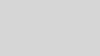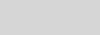# Project Austin Part 2 of 6: Page CurlingEric Brumer - MSFT

Hi, my name is Eric Brumer. I’m a developer on the C++ compiler optimizer, but I’ve spent some time working on Project Code Name Austin to help showcase the power and performance of C++ in a real-world program. For a general overview of the project, please check out the original blog post. The source code for Austin, including the bits specific to page curling described here, can be downloaded on CodePlex.

In this blog post, I’ll explain how we implemented page turning in the “Full page” viewing mode. We wanted to make flipping through the pages in Austin to feel like flipping through pages in a real book. To that end, we built on some existing published work to achieve performant and realistic page curling.

Before going further, take a look at a video of page curling in action!

Realistic page curling

A brilliant paper by Hong et. al. called “Turning Pages of 3D Electronic Books” claims that turning a page of a physical book can be simulated as deforming a page around a cone.  See  for the details.

Here’s a (poorly drawn) diagram to help explain the concept in the paper. The flat sheet of paper is deformed around the cone to simulate curling. By changing the shape and position of the cone you can simulate more or less curling.Similarly, you can also curl a flat sheet of paper around a cylinder. Here’s another (poorly drawn) diagram to help explain that concept.To simulate curling, we use a combination of curling around a cone and curling around a cylinder:

• If the user is trying to curl from the top-right of the page, we simulate pinching the top right corner of a piece of paper by deforming around a cone.
• If the user is trying to curl from the center-right of the page, we simulate pinching the center of a piece of paper by deforming around a cylinder.
• If the user is trying to curl from the bottom-right of the page, we simulate pinching the bottom right corner of a piece of paper by deforming around the cone flipped upside down.

Anywhere in between and we use a combination of cone & cylinder deforming.

Some geometry

Here are the details to transform a page around a cylinder. There is similar geometry to transform a page to a cylinder described in . Given the point Pflat with coordinates {x1, y1, z1 = 0} of a flat page, we want to transform it into Pcurl with coordinates {x2, y2, z2} the point on a cylinder with radius r that is lying on the ‘spine’ of the book. Consider the following diagram. Note the x & z axes (the y axis is in & out of your computer screen). Also keep in mind I am representing the flat paper & cylinder using the same colors as in the diagrams above.The key insight is that the distance from the origin to Pflat (x1) is the same arc distance as from the origin to Pcurl along the cylinder. Then, from simple geometry, we can say that β = x1 / r. Now, to get Pcurl, we take the origin, move it down by ‘r’ on the z axis, rotate about β, then move it up by ‘r’ on the z axis. So, the math ends up being:The above equations compute Pcurl by wrapping a flat page around cylinder.   contains the equations to compute a different Pcurl by wrapping a flat page around a cone. Once we compute both Pcurl values, we combine the results based on where the user is trying to curl the page. Lastly, after we have computed the two curled points, we rotate the entire page about the spine of the book.

The specific parameters are tuned by hand: the cone parameters, the cylinder width, and the rotation about the spine of the book.

Code

The source code for Austin, including the bits specific to page curling described here, can be downloaded on CodePlex. The page curling transformation is done in journal/views/page_curl.cpp, specifically in page_curl::curlPage(). The rest of the code in that file is to handle uncurling pages (forwards or backwards) when the user lifts their finger off the screen. I’m omitting some important details, but this code gives the rough idea.

for (b::int32 j = 0; j < jMax; j++)
{
…

for (b::int32 i = 0; i < iMax; i++)
{

float coneX = x;
float coneY = y;
float coneZ = z;
{
// Compute conical parameters coneX, coneY, coneZ
…
}

float cylX = x;
float cylY = y;
float cylZ = z;
{
float beta = cylX / cylRadius;

// Rotate (0,0,0) by beta around line given by x = 0, z = cylRadius.
cylX = -cylZ * sin(beta);
cylZ = cylZ * cos(beta);

// Then rotate by angle about the y axis
cylX = cylX * cos(angle) – cylZ * sin(angle);
cylZ = cylX * sin(angle) + cylZ * cos(angle);

// Transform coordinates to the page
cylX = (cylX * pageCoordTransform) – pageMaxX;
cylY = (-cylY * pageCoordTransform) + pageMaxY;
cylZ = cylZ * pageCoordTransform;
}

// combine cone & cylinder systems
x = conicContribution * coneX + (1-conicContribution) * cylX;
y = conicContribution * coneY + (1-conicContribution) * cylY;
z = conicContribution * coneZ + (1-conicContribution) * cylZ;

vertexBuffer[jOffset + i].position.x = x;
vertexBuffer[jOffset + i].position.y = y;
vertexBuffer[jOffset + i].position.z = z;
}
}

Automatic Vectorization

A new feature in the Visual Studio 2012 C++ compiler is automatic vectorization. The C++ compiler analyzes loop bodies and generates code targeting the SSE2 instruction set to take advantage of CPU vector units. For an introduction to the auto vectorizer, and plenty of other information, please see the vectorizer blog series.

The inner loop above is vectorized by the Visual Studio 2012 C++ compiler. The compiler is able to vectorize all of the transcendental functions in math.h, along with the standard arithmetic operations (addition, multiplication, etc). The generated code loads four values of x, y, and z. Then it computes four values of cylX, cylY, cylZ at a time, computes curlX, curlY, curlZ at a time, and stores the result into the vertex buffer for four vertices.

I know the code gets vectorized because I specified the /Qvec-report:1 option in my project settings, under Configuration Properties -> C/C++ -> Command Line, as per the following picture:Then, after compiling, the output window shows which loops were vectorized, as per the following picture:Eric’s editorial: we decided late during the product cycle to include the /Qvec-report:1 and /Qvec-report:2 switches, and we did not have time to include them in the proper menu location.

If you do not see a loop getting vectorized and wonder why, you can specify the /Qvec-report:2 option. We offer some guidance on handling loops that are not vectorized in a vectorizer blog post.

Because of the power of CPU vector units, the ‘i’ loop gets sped up by a factor of 1.75. In this instance, we are able to compute Pcurl (the combination of cone & cylinder) for four vertices at a time. This frees up CPU time for other rendering tasks, such as shading the page.

Performance

To curl a single page, we need to calculate Pcurl for each vertex comprising a piece of paper. To my count, this involves 4 calls to sin, 3 calls to cos, 1 arcsin, 1 sqrt, and a dozen or so multiplications, additions and subtractions – for each vertex in a piece of paper – for each frame that we are rendering!

We aim to render at 60fps, which means we have around 15 milliseconds to curl the pages vertices and render them — otherwise the app will feel sluggish. With this loop getting auto vectorized, we’re able to free up CPU time for other rendering tasks, such as shading the page.

References

 L. Hong, S.K. Card,  and J. Chen,   “Turning Pages of 3D Electronic Books”, in Proc. 3DUI, 2006, pp.159-165.

Posted in C++

## 1 comment

•